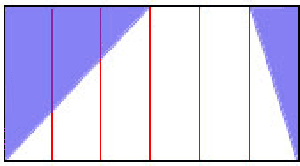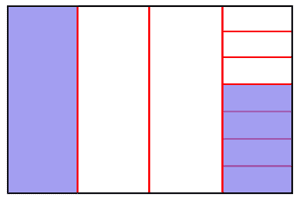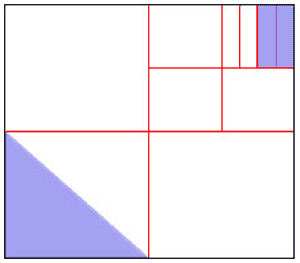# Fraction Figures

Part 1: Use basic fraction operations to describe the fraction of each of the following figures that is shaded. For this investigation, you can assume that pieces that look the same size are the same size.

a)b)c)Part 2: Draw a figure that models 1/2 * 1/4 + 1/3 * 3/8

(Source: Adapted from Mathematics for Elementary Teachers, Sybilla Beckman, 2nd ed., 2008)